# Bomb Lab

This is the fourth experiment of assembly language. I didn’t expect to use the well-known bomb lab on CSAPP. It is also very powerful.

# Turn the bomb into a “dumb bomb”

As a perfectionist of the glass heart, naturally, I don’t want my own explosive record to be registered, so I have to find a way to debug the bomb locally. Fortunately, both sides are x86, at least binary files can run. Running in the local linux environment, error:

Initialization error: Running on an illegal host 


After observing the disassembly, and giving the c file a line like this:

/* Do all sorts of secret stuff that makes the bomb harder to defuse. */
initialize_bomb();


Found that this function is checking the runtime environment, it is not a good thing to look at its comments. Replace the function call statement with a null instruction with a binary editor: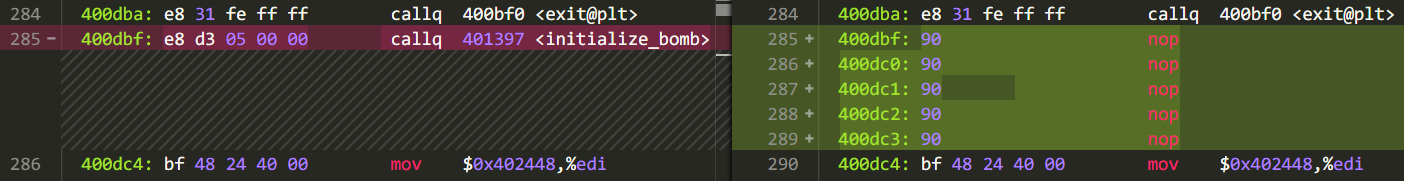Then you can run it locally, but the information about the bomb explosion will still be sent out (it was accidentally bombed during class) and continue to hack —

The bomb explosion will call the explode_bomb function, and the phase_defused function will be called when the bomb is removed. (It is really conscience to keep the symbol table when compiling, which greatly reduces the difficulty); both functions call a function called send_msg to pass the information. Therefore, you only need to replace the function call statement in explode_bomb and phase_defused with a null instruction (the function has a return value, but it has not been used, so it is not investigated in detail):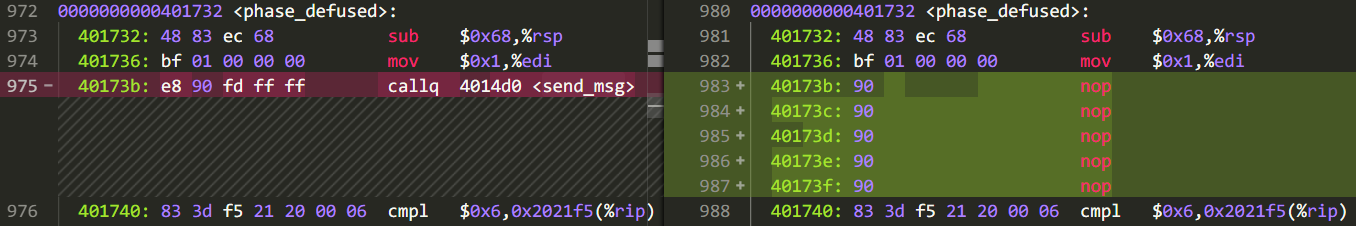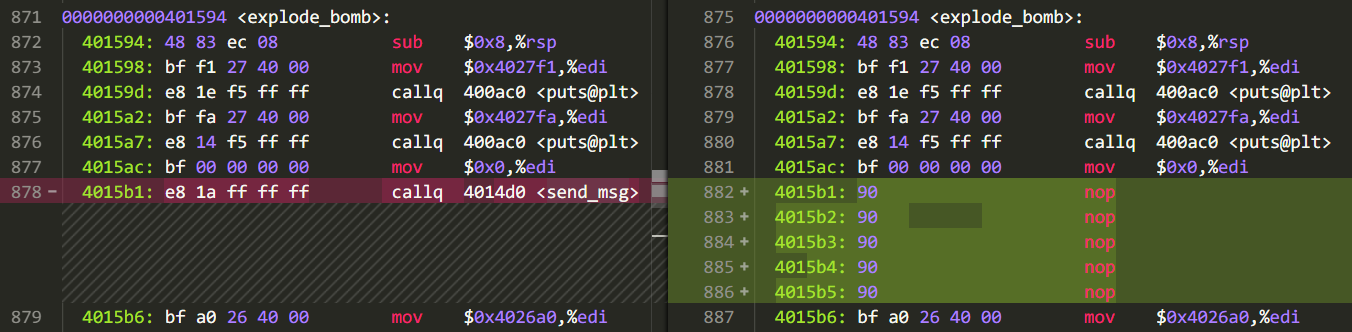You’re done, and at this point the bomb has become a dumb bomb.

# Tool introduction

If a worker wants to do something good, he must first sharpen his tools. The following tools were mainly used in this experiment:

ToolEffect
gdbDynamic debugging
objdumpDisassembly
Hex Editor NeoBinary Editing

# Phase 1

The first question is very simple. The topic implements the strings_not_equal function. Its function is just like its name. It only needs to know the incoming parameters in rdi, rsi, and the return value is eax. Find another string to participate in the comparison based on the address Verbosity leads to unclear, inarticulate things.

# Phase 2

The second problem, there is a function read_six_numbers, the function is to read six numbers from the string pointed to by the first parameter, stored in the array pointed to by the second int pointer. Note that when the function calls sscanf, the parameters are more than seven, and the parameters are passed using the stack. This point is not realized by the decompilation tool IDA.

After reading the six numbers, the program confirms that the first item is 0, the second item is 1, and then follows the law of Fibonacci numbers. Each item is the sum of the first two items, so the answer is:

0 1 1 2 3 5


This shows the IDA interface, which can display blocks separated by jump instructions, which greatly improves the readability of the code: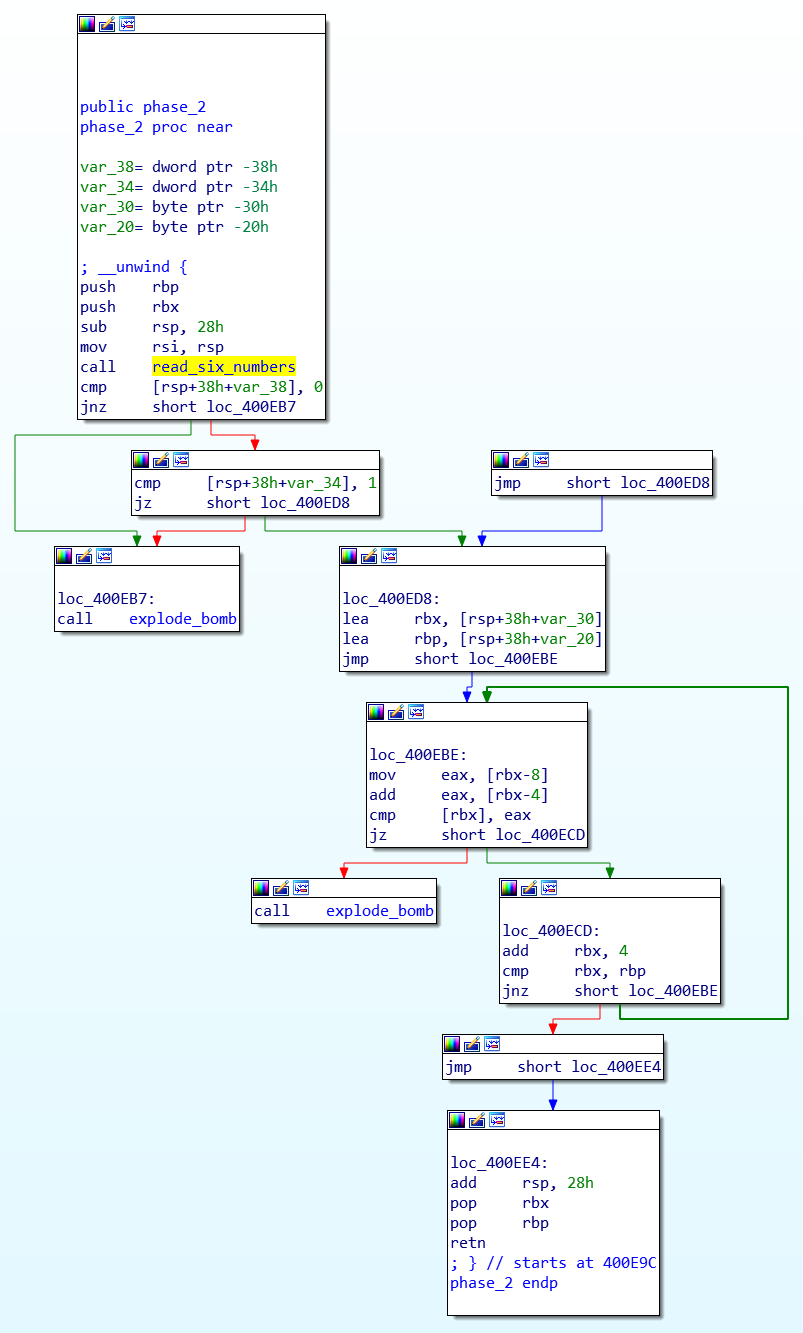# Phase 3

The third question, the jump table, is equivalent to a switch statement. The input string is "%d %c %d", with the first number as the index, there are 0 to 7 kinds of choices, each of which has corresponding characters and numbers. Here, for convenience, the first number is 0, and the character corresponding to 79H is y. The number 210H is 528. So a possible answer: 0 y 528

# Phase 4

The input to this level is the two numbers a, b, and the conditions for the bombing are a == func4(7, b) and 2 <= b <= 4.

By studying the function body of func4, it is known that this is a recursive function, and its logical equivalent python function is:

def func4(x, y):
if x <= 0:
return 0
if x == 1:
return y
return func4(x-1, y) + func4(x-2, y) + y


Taking b = 3, we can calculate func4(7, 3) = 99, so the answer is 99 3

# Phase 5

This question requires reading a string with a length of 6. Assuming one of the characters is c, the following program loops from 1 to 6, adding array_3154[c & 0xF] the character at it to an empty string s. The length of the array is exactly 16, and the memory is checked to see what it is maduiersnfotvbyl. Finally, "flyers" compares with the target string . Therefore, construct a string so that the last four digits of each character are the index of the corresponding position of the flyer. See below:yonuvw

# Phase 6

The sixth question is really too much. . .

First read in six numbers (or read_six_numbers function), then the double loop determines that the six numbers are different. (The full arrangement of the six numbers is 720. It is also possible to find a violent search.)

There are still two loops that look a bit embarrassing, and can only be dynamically debugged with gdb.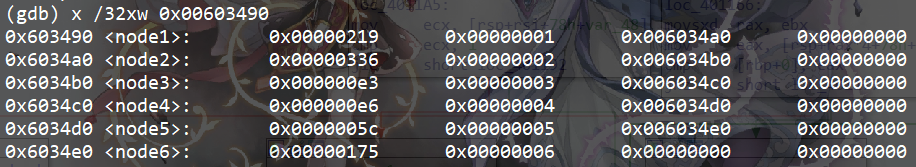The above figure is an array frequently involved in the loop. After observing, the program is newly opened with an array corresponding to 0x603490 to 0x6034e0 according to the original 1 to 6. After comparing the positions in the array shown in the first column of the above figure, they must be in descending order. Therefore, the numbers shown in the first column of the above figure are arranged, and then the order corresponding to each number (the second column in the above figure) is used as the answer.

2 1 6 4 3 5


# Secret Phase

The existence of hidden gates is known from various places, such as comments in the c file, and the leaderboards that have cracked the seven levels.

Looking directly at the function list reveals that there is a function called secret_phase. Use gdb to return 0;, add a breakpoint before the statement, use the call secret_phasecommand after the first six levels of cracking to enter the hidden off. If the hidden off is called directly, the leaderboard will display phase 1 invalid.

The last level is relatively simple. Read a decimal number y. We want the return value of the fun7 function to be 0.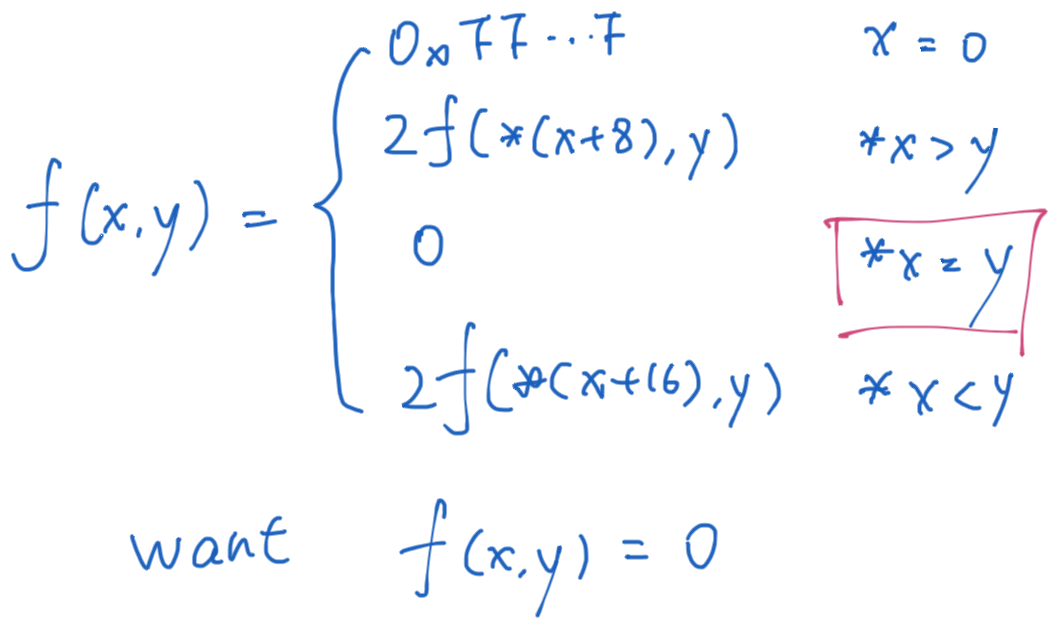Although the definition of the function is rather strange, it can be seen that if you want the function to return a value of 0, you only need to make y equal to the number pointed to by the pointer of the first parameter. You can see that the number is 36 by looking at the memory.